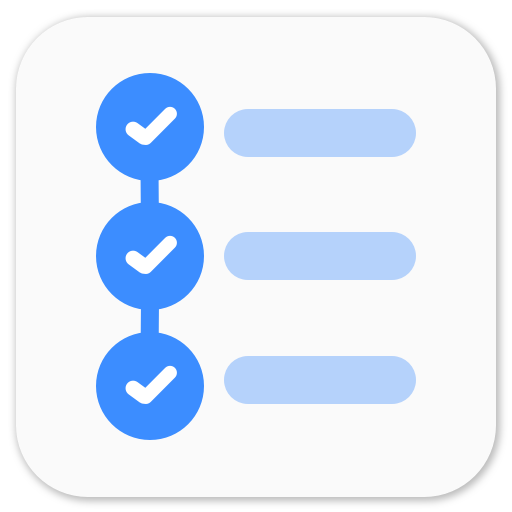Taskito - Calendar based to-do listKalman Filter is a set of mathematical equations that provides an efficient computational (recursive) means to estimate the state of a process, in a way that minimizes the mean of the squared error. It uses a series of measurements observed over time, containing noise (random variations) and other inaccuracies, and produces estimates of unknown variables that tend to be more precise than those that would be based on a single measurement alone.The filter is named for Rudolf (Rudy) E. Kálmán, one of the primary developers of its theory.

The algorithm works in a two-step process: in the prediction step, the Kalman filter produces estimates of the current state variables, along with their uncertainties. Because of the algorithm’s recursive nature, it can run in real time using only the present input measurements and the previously calculated state; no additional past information is required.

Since Kalman filter is a recursive estimator, it needs only the estimated state from the previous time step and the current measurement to compute the estimate for the current state. In contrast to batch estimation techniques, no history of observations and/or estimates is required. This can be very helpful to improve tracking of the objects. I implemented Kalman Filter in SimpleCV. As of now, I’m just predicting the center of the object using its current and previous centers. I might add more features in Kalman Filter later viz pixel velocity, real time velocity, areaRatio, etc.

Kalman Filter with OpenCV: I tried using OpenCV 2.4 version to implement Kalman Filter, but it turns out that the bindings are incomplete. see here. As of now it’s not possible to implement Kalman Filter using cv2. So, cv it is.

Create Kalman Filter

import cv2.cv as cv
kalman = cv.CreateKalman(4, 2, 0)
kalman_state = cv.CreateMat(4, 1, cv.CV_32FC1)
kalman_process_noise = cv.CreateMat(4, 1, cv.CV_32FC1)
kalman_measurement = cv.CreateMat(2, 1, cv.CV_32FC1)

Kalman filter is now ready to be used.

Set Kalman Filter

# set previous state for prediction
kalman.state_pre[0,0]  = x
kalman.state_pre[1,0]  = y
kalman.state_pre[2,0]  = 0
kalman.state_pre[3,0]  = 0

# set kalman transition matrix
kalman.transition_matrix[0,0] = 1
kalman.transition_matrix[0,1] = 0
kalman.transition_matrix[0,2] = 0
kalman.transition_matrix[0,3] = 0
kalman.transition_matrix[1,0] = 0
kalman.transition_matrix[1,1] = 1
kalman.transition_matrix[1,2] = 0
kalman.transition_matrix[1,3] = 0
kalman.transition_matrix[2,0] = 0
kalman.transition_matrix[2,1] = 0
kalman.transition_matrix[2,2] = 0
kalman.transition_matrix[2,3] = 1
kalman.transition_matrix[3,0] = 0
kalman.transition_matrix[3,1] = 0
kalman.transition_matrix[3,2] = 0
kalman.transition_matrix[3,3] = 1

# set Kalman Filter
cv.SetIdentity(kalman.measurement_matrix, cv.RealScalar(1))
cv.SetIdentity(kalman.process_noise_cov, cv.RealScalar(1e-5))
cv.SetIdentity(kalman.measurement_noise_cov, cv.RealScalar(1e-1))
cv.SetIdentity(kalman.error_cov_post, cv.RealScalar(1))

Predict new points using Kalman filter

kalman_prediction = cv.KalmanPredict(kalman)
predict_pt  = (kalman_prediction[0,0], kalman_prediction[1,0])

Kalman Correction

kalman_estimated = cv.KalmanCorrect(kalman, kalman_measurement)
state_pt = (kalman_estimated[0,0], kalman_estimated[1,0])

Changing Kalman Measurement

kalman_measurement[0, 0] = x
kalman_measurement[1, 0] = y

Pseudo Code

Create Kalman Filter
Start Tracking
While (some condition)
x, y = track()
Set Kalman Filter
Change Kalman Measurements
Predict Kalman
Kalman Correction
Update the center of the object

This was the video where I use Kalman Filter to predict the center of the objects. I am not using Kalman Correction as of now. I have implemented Kalman Filter in SimpleCV Tracking Feature. You can find my GitHub SimpleCV Kalman branch here.

Using Kalman Filter with SimpleCV

from SimpleCV import *
def camshift():
cam = Camera()
img = cam.getImage()
d = Display(img.size())
bb1 = getBB() # Get Bounding Box from some method
fs1=[]
while True:
try:
img1 = cam.getImage()
fs1 = img1.track("camshift",fs1,img,bb1,num_frames=5)
fs1.drawBB()
fs1.draw()
fs1.drawPredict(color=Color.RED)
camshift()

So, this is it. Kalman Filter.

P.S. Going back to the campus in few days. quite excited.

Playing around with Android UI

Articles focusing on Android UI - playing around with ViewPagers, CoordinatorLayout, meaningful motions and animations, implementing difficult customized views, etc.